# COMP 1321 Digital Infrastructure Richard Henson University of

• Slides: 29
Download presentationCOMP 1321 Digital Infrastructure Richard Henson University of Worcester October 2012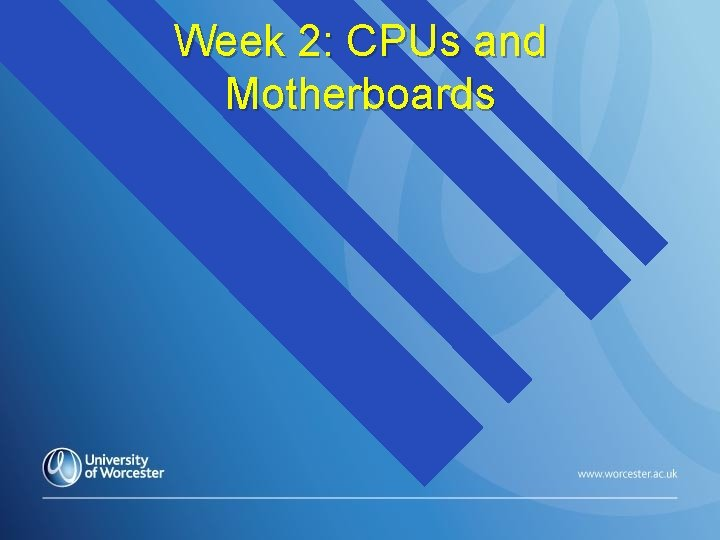Week 2: CPUs and Motherboards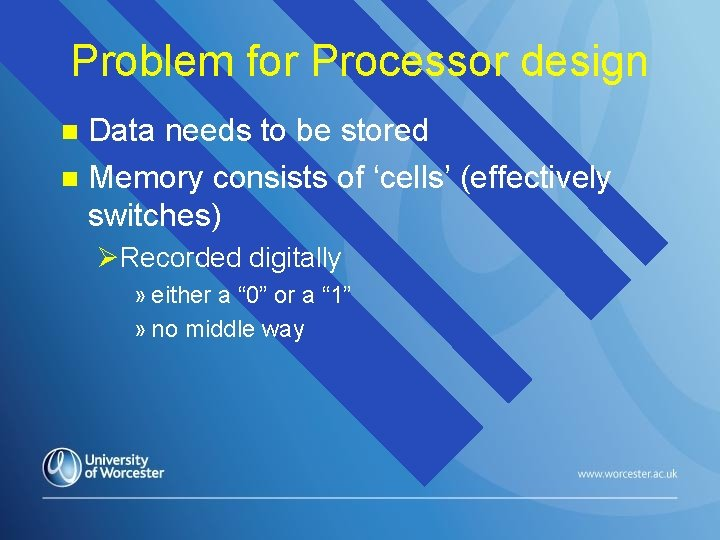Problem for Processor design Data needs to be stored n Memory consists of ‘cells’ (effectively switches) n ØRecorded digitally » either a “ 0” or a “ 1” » no middle way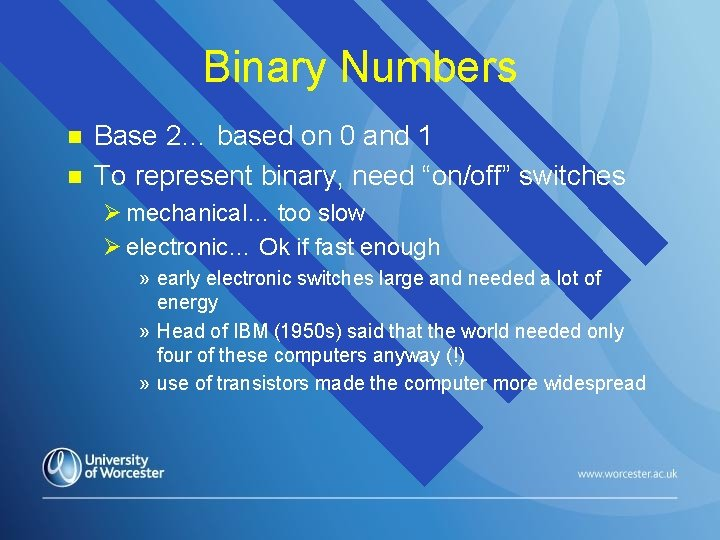Binary Numbers n n Base 2… based on 0 and 1 To represent binary, need “on/off” switches Ø mechanical… too slow Ø electronic… Ok if fast enough » early electronic switches large and needed a lot of energy » Head of IBM (1950 s) said that the world needed only four of these computers anyway (!) » use of transistors made the computer more widespread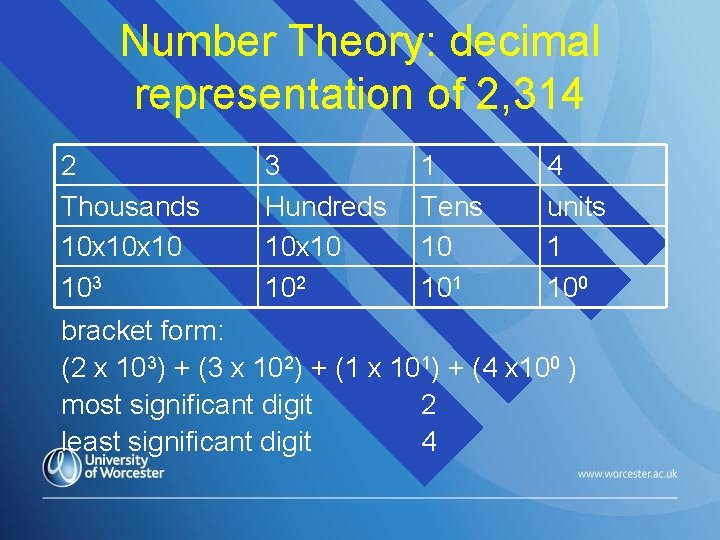Number Theory: decimal representation of 2, 314 2 Thousands 10 x 10 103 3 Hundreds 10 x 10 102 1 Tens 10 101 4 units 1 100 bracket form: (2 x 103) + (3 x 102) + (1 x 101) + (4 x 100 ) most significant digit 2 least significant digit 4Some definitions… binary digit bit 0 or 1 n byte: a group of 8 bits n (nibble: a group of 4 bits) n word: a group of bits of a fixed length (actual length of a word is rather arbitrary) n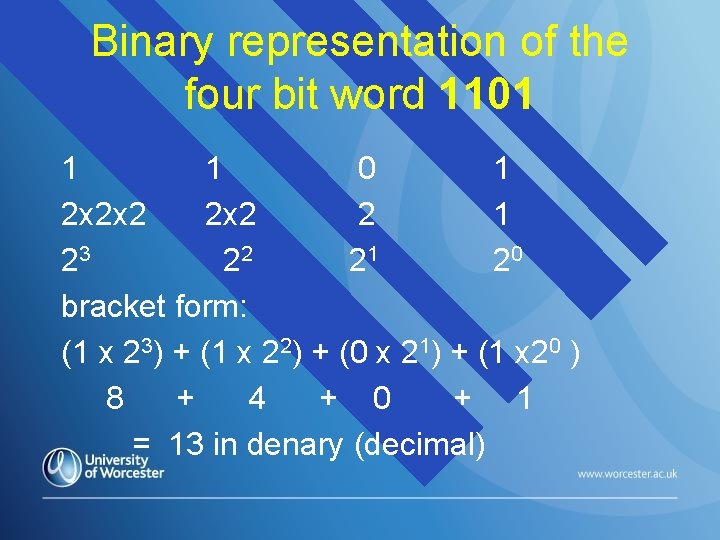Binary representation of the four bit word 1101 1 1 0 1 2 x 2 x 2 2 1 23 22 21 20 bracket form: (1 x 23) + (1 x 22) + (0 x 21) + (1 x 20 ) 8 + 4 + 0 + 1 = 13 in denary (decimal)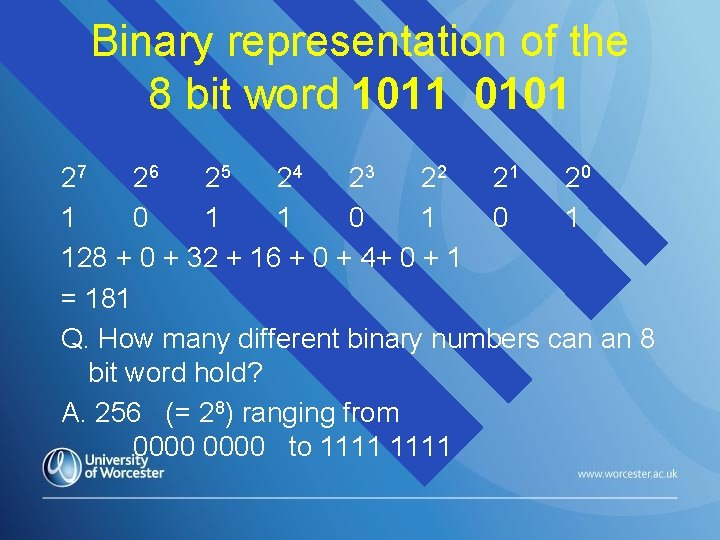Binary representation of the 8 bit word 1011 0101 27 26 25 24 23 22 21 20 1 0 1 0 1 128 + 0 + 32 + 16 + 0 + 4+ 0 + 1 = 181 Q. How many different binary numbers can an 8 bit word hold? A. 256 (= 28) ranging from 0000 to 1111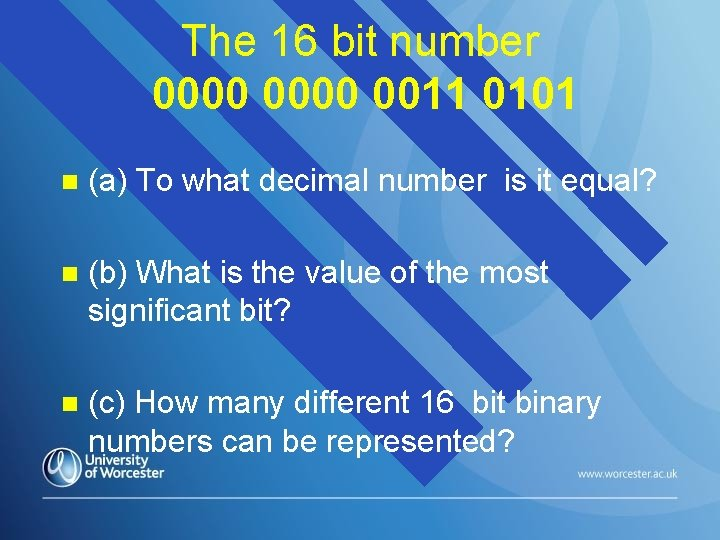The 16 bit number 0000 0011 0101 n (a) To what decimal number is it equal? n (b) What is the value of the most significant bit? n (c) How many different 16 bit binary numbers can be represented?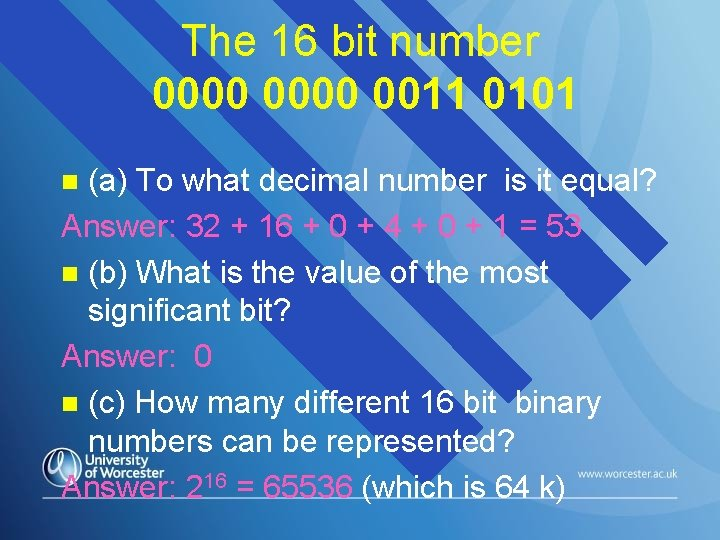The 16 bit number 0000 0011 0101 (a) To what decimal number is it equal? Answer: 32 + 16 + 0 + 4 + 0 + 1 = 53 n (b) What is the value of the most significant bit? Answer: 0 n (c) How many different 16 bit binary numbers can be represented? Answer: 216 = 65536 (which is 64 k) n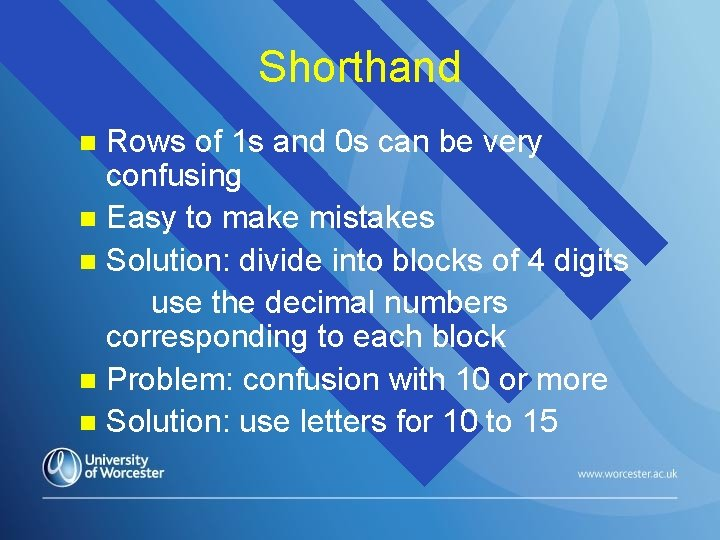Shorthand Rows of 1 s and 0 s can be very confusing n Easy to make mistakes n Solution: divide into blocks of 4 digits use the decimal numbers corresponding to each block n Problem: confusion with 10 or more n Solution: use letters for 10 to 15 nHexadecimal notation Decimal 0 1 2 3 4 5 6 7 8 9 Binary Hexadecimal 0000 0001 0010 0011 0100 0101 0110 0111 1000 1001 0 1 2 3 4 5 6 7 8 9Hexadecimal notation Decimal 10 11 12 13 14 15 Binary 1010 1011 1100 1101 1110 1111 Hexadecimal A B C D E FNotation n Useful to know what type of number we are dealing with e. g. “ 110” Øuse subscript at the end Ø 11010 = 110 (denary) Ø 1102 = 110 (binary) = 6 (denary) Ø 110 H or 11016 = 110 (hexadecimal) = 272 (denary) n So now you know!Now, let’s make a Computer … at least the CPU … Pentium 4 Ultra Sparc 1 Pentium 3 Opteron Itanium 2 Mc. Kinley 21364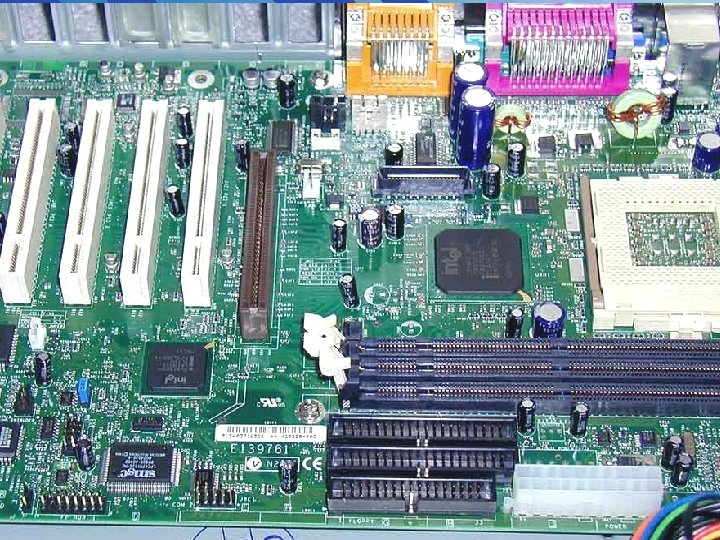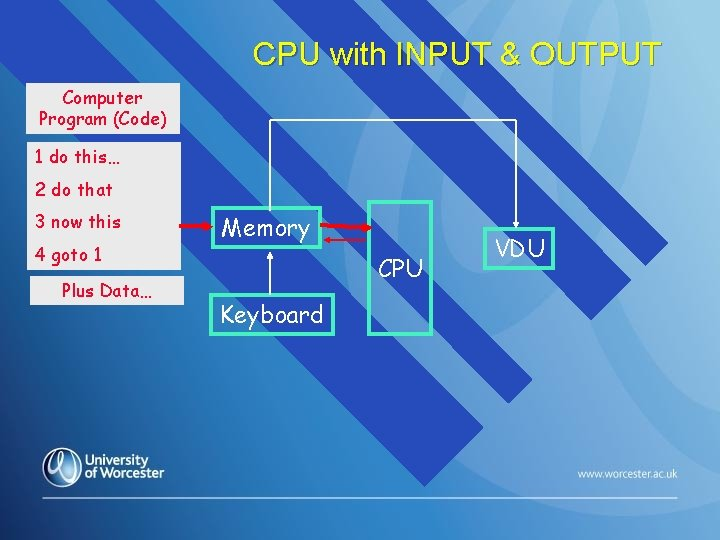CPU with INPUT & OUTPUT Computer Program (Code) 1 do this… 2 do that 3 now this 4 goto 1 Plus Data… Memory CPU Keyboard VDU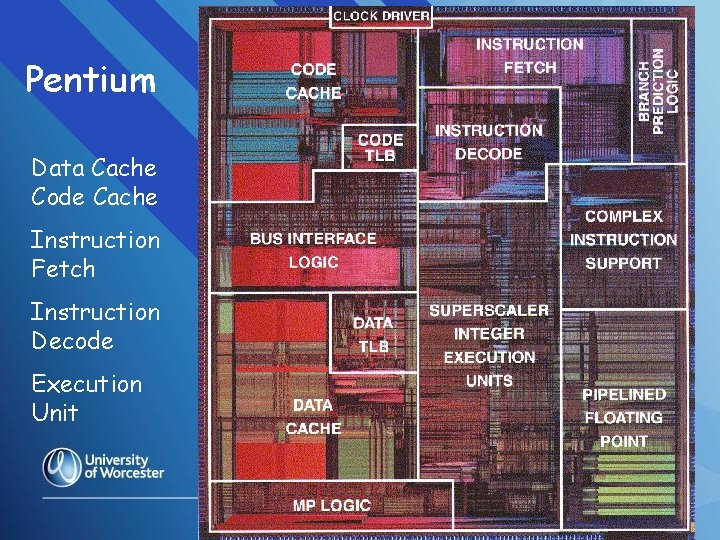Pentium Data Cache Code Cache Instruction Fetch Instruction Decode Execution Unit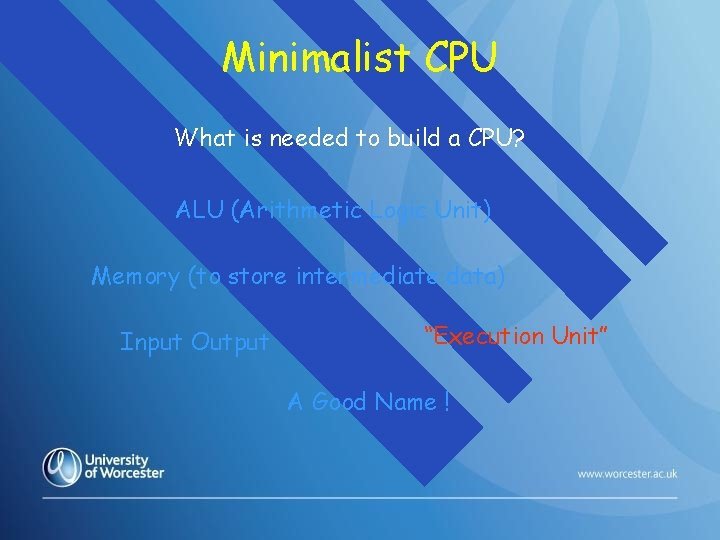Minimalist CPU What is needed to build a CPU? ALU (Arithmetic Logic Unit) Memory (to store intermediate data) Input Output “Execution Unit” A Good Name !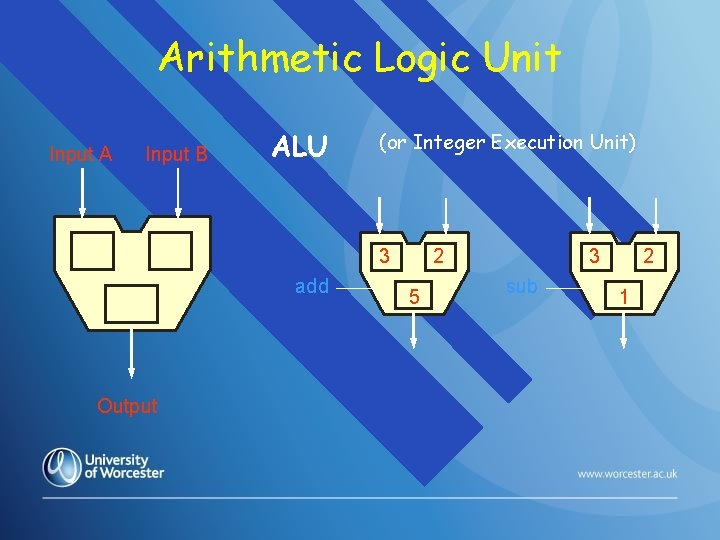Arithmetic Logic Unit Input A Input B ALU (or Integer Execution Unit) 3 add Output 2 5 3 sub 2 1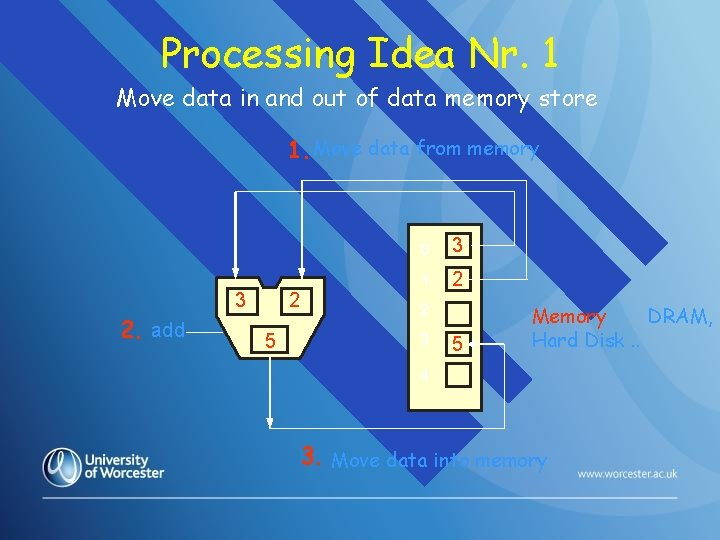Processing Idea Nr. 1 Move data in and out of data memory store 1. Move data from memory 3 2. add 2 5 0 3 1 2 2 3 5 Memory DRAM, Hard Disk. . 4 3. Move data into memoryProcessing Idea Nr. 2 Move instructions into CPU from code memory Instruction Memory (Code Memory) mov 3 in from memory 3 mov 2 in from memory IP add the two numbers mov the result to memory Program add 2 5 0 3 1 2 2 3 4 5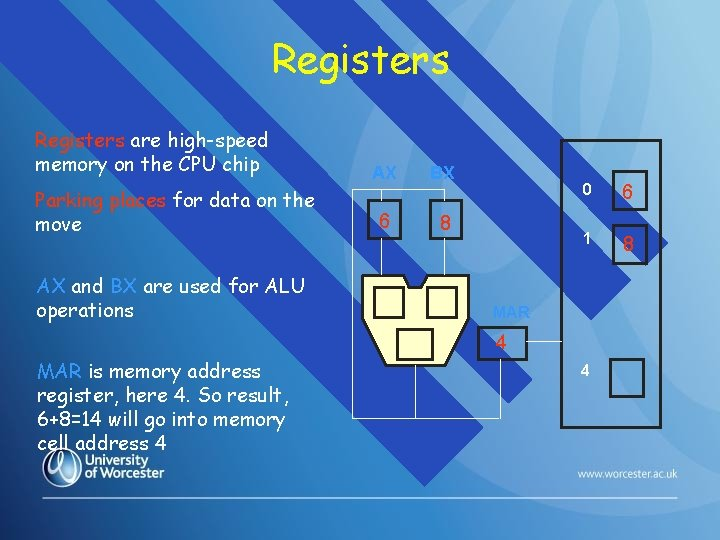Registers are high-speed memory on the CPU chip Parking places for data on the move AX and BX are used for ALU operations AX BX 6 8 0 6 1 8 MAR 4 MAR is memory address register, here 4. So result, 6+8=14 will go into memory cell address 4 4Our computer so far … 0 1 Instruction Memory Data Memory mar ip 4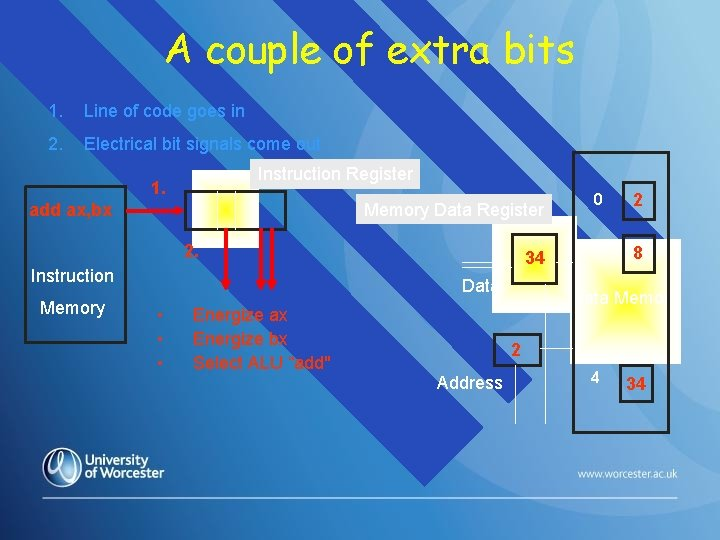A couple of extra bits 1. Line of code goes in 2. Electrical bit signals come out Instruction Register 1. Memory Data Register add ax, bx 2. Instruction Memory 34 Data • • • Energize ax Energize bx Select ALU “add" 0 2 1 8 Data Memory 2 Address 4 34Moving data into Registers For example … mov ax ,  mov bx ,  Instruction Memory AX BX 8 7 mar 0 5 1 8 2 7 3 6 4 1 mov ax ,  mov bx ,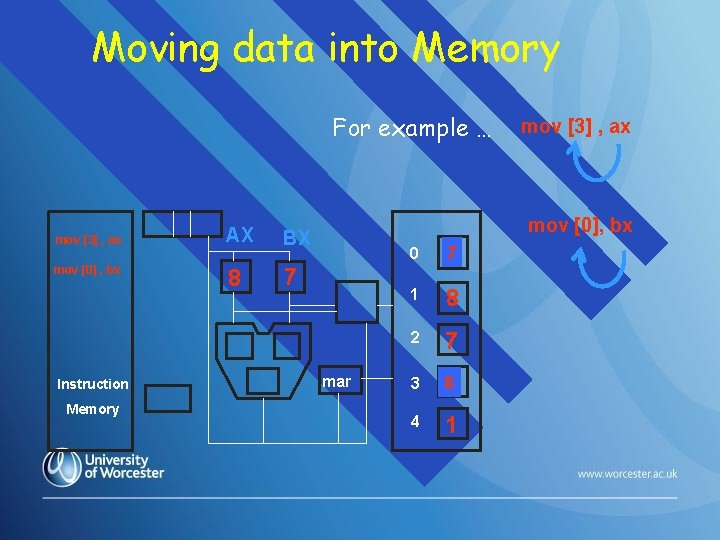Moving data into Memory For example … mov  , ax mov  , bx Instruction Memory AX BX 8 7 mov  , ax mov , bx mar 0 7 5 1 8 2 7 3 8 6 4 1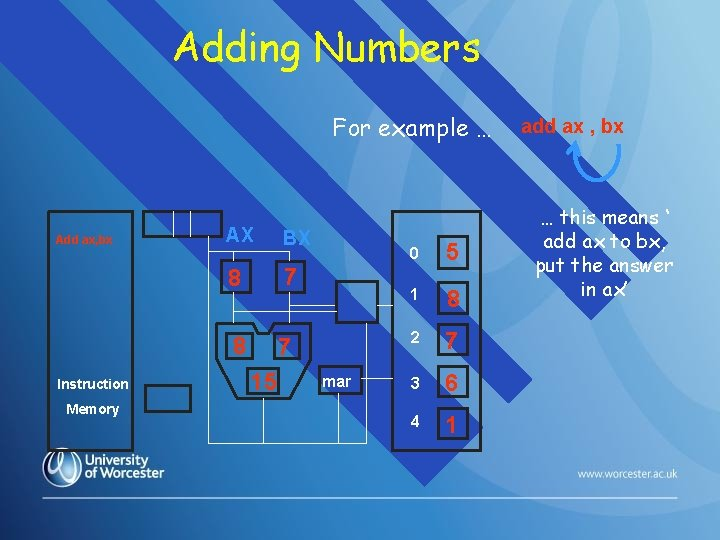Adding Numbers For example … Add ax, bx Instruction Memory AX BX 8 7 15 mar 0 5 1 8 2 7 3 6 4 1 add ax , bx … this means ‘ add ax to bx, put the answer in ax’So THAT’S how it works! Next week: the programming!